# Series and Parallel Resistors

We present examples of circuits with series and parallel resistors and the formulas to calculate the equivalent resistance of these groups of resistors.

## Resistors in Series

The resistors R1, R2 ...., Rm in the circuit on the left side are said to be in series because the same current passes through them. They behave in the same way as the circuit on the right of resistance Req given by the sum of the resistances R1, R2 and R3.

Req = R1 + R2 ....+ Rm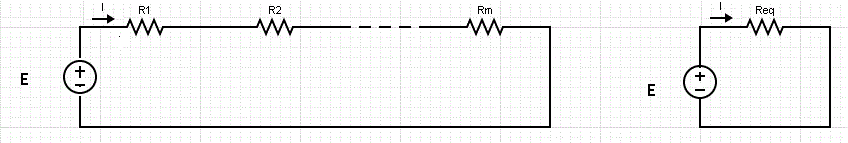The current I in the above circuit is given by
I = E / Req

## Resistors in Parallel

The voltage across each of the resistors R1, R2 ...., Rm in the circuit on the left is the same and therefore these resistors are said to be in parallel. They behave in the same way as the circuit on the right of resistance Req that is given by the equation:

1 / Req = 1 / R1 + 1 / R2 + .... + 1 / Rm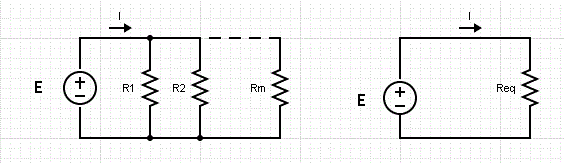## Resistors in Series: Examples with Detailed Solutions

Example 1
Find the current I passing through and the voltage across each of the resistors in the circuit below.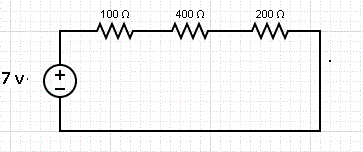The three resistor in series have a resistance Req given by the sum of the three resistances. Hence
Req = 100 + 400 + 200 = 700 Ω
The current I passing through R1, R3 and R3 is the same and is calculated as follows:
I = 7 v / 700 Ω = 0.01 A
The voltage across each resistance is calculated using
Ohm's law as follows:
The voltage across 100Ω: VR1 = 100 × I = 100 × 0.01 = 1 v
The voltage across 400Ω: VR2 = 400 × I = 400 × 0.01 = 4 v
The voltage across 200Ω: VR3 = 200 × I = 200 × 0.01 = 2 v

## Resistors in Parallel: Examples with Detailed Solutions

Example 2
Find current I in the circuit below and the current passing through each of the resistors in the circuit.Solution to Example 2
The three resistors are in parallel and behave like a resistor with resistance Req given by
1 / Req = 1 / 100 + 1 / 400 + 1 / 200
Multiply all terms by 400 and simplify to obtain
400 / Req = 4 + 1 + 2
Solve for Req to obtain
Req = 400 / 7 Ω
The main current I is given by
I = 7 / Req = 7 / (400 / 7) = 49 / 400 A
We now use Ohm's law to find the current passing through each resistor.
The current through the resistor of 100 Ω: I1 = 7 / 100 A
The current through the resistor of 400 Ω: I2 = 7 / 400 A
The current through the resistor of 200 Ω: I3 = 7 / 200 A
As an exercise; check that the sum of the three currents above is equal to the current I = 49 / 400 A.

## Series and Parallel Resistors: Examples with Detailed Solutions

Example 3
Find current I in the circuit below.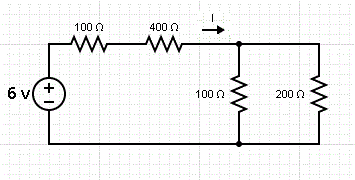Solution to Example 3
The two resistors that are in series are grouped as Req1 in the equivalent circuit below and their resistance is given by the sum
Req1 = 100 + 400 = 500 Ω
The two resistors that are in parallel are grouped as Req2 in the equivalent circuit below and their resistance is given by the equation
1 / Req2 = 1 / 100 + 1 / 200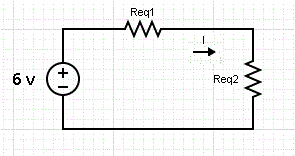Solve to obtain
Req2 = 200 / 3 Ω
Req1 and Req2 are in series and therefore are equivalent to R given by the sum
R = Req1 + Req2 = 500 + 200 / 3 = 1700 / 3 Ω
We now use Ohm's law to find current I.
I = 6 / R = 6 / (1700 / 3) = 18 / 1700 A

Example 4
What resistance x in parallel with resistances 100 Ω and 200 Ω gives an equivalent resistance of 50 Ω?
Solution to Example 4
Let x be the resistance to be found. The equivalent resistance of the all three resistor in parallel is known. We use the equation that gives the equivalent resistance of resistors in parallel as follows
1 / 50 = 1 / 100 + 1 / 200 + 1 / x
which gives
1 / x = 1 / 50 - 1 / 100 - 1 / 200
Set all fractions on the right to the common denominator 200 and rewrite the above equation as
1 / x = 4 / 200 - 2 / 200 - 1 / 200 = 1 / 200
solve for x to obtain
x = 200 Ω

Example 5
Show that if the resistors with resistances R1, R2 ,..., Rm are in parallel, then the equivalent resistance Req is always smaller than R1, R2, ..., Rm.
Solution to Example 5
The equivalent resistance Req is given by the equation
1 / Req = 1 / R1 + 1 / R2 + ... + 1 / Rm
Since R1, R2, ... Rm are positive quantities, we can write that
1 / Req > 1 / Ri , where Ri is any of the resistances.
Multiply all terms of the inequality above by (Req × Ri) and simplify to obtain
Ri > Req
or
Req < Ri , i = 1, 2, ... m.

## More References and Links

Ohm's Law with Examples
Free SAT II Physics Practice Solutions on DC Electric Circuits